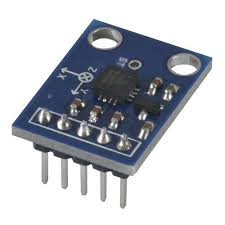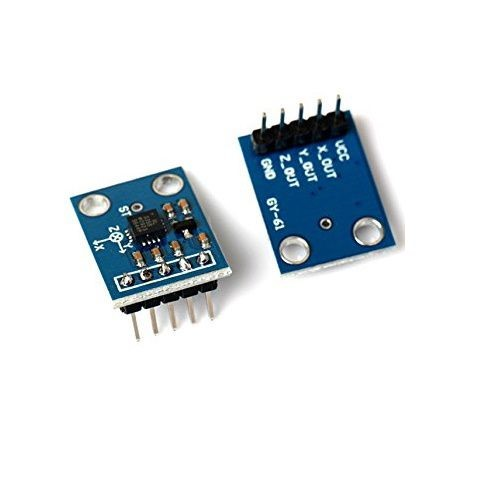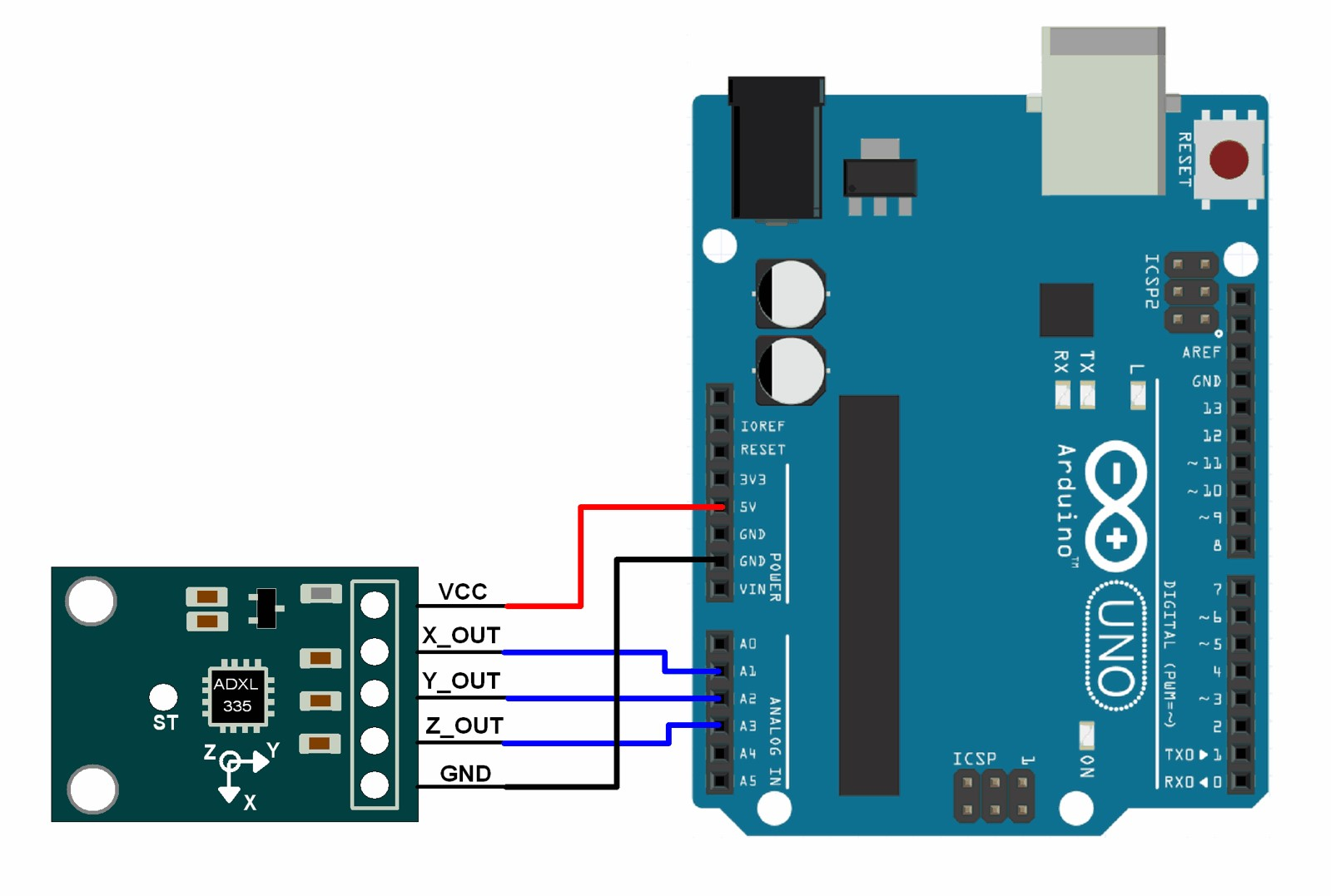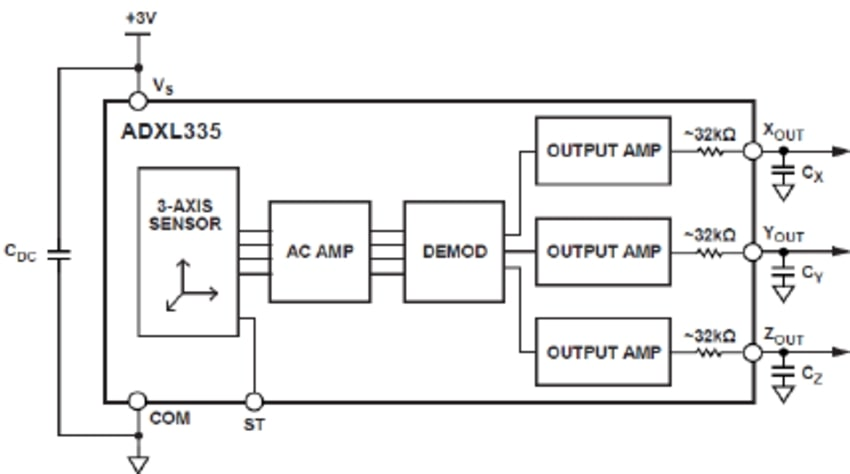Accelerometer

Description:

This Accelerometer module is based on the popular ADXL335 three-axis analog accelerometer IC, which reads off the X, Y and Z acceleration as analog voltages. By measuring the amount of acceleration due to gravity, an accelerometer can figure out the angle it is tilted at with respect to the earth. By sensing the amount of dynamic acceleration, the accelerometer can find out how fast and in what direction the device is moving.

Working Principle:

Accelerometers use the piezoelectric effect – they contain microscopic crystal structures that get stressed by accelerative forces, which causes a voltage to be generated. Another way to do it is by sensing changes in capacitanceTypes of Accelerometer sensor:• VCC :  To  be connected to 5v supply
• X : To be connected to Analog Pin A5
• Y: To be connected to Analog Pin A4
• Z: To be connected to Analog Pin A4
• GND : To be connected to ground

Arduino Code:

int x;

int y;

int z;

void setup()

{

Serial.begin(9600);  // opens serial port, sets data rate to 9600 bps

}

void loop()

{

Serial.print(“X = “);  // print x adc value

Serial.println(x);

Serial.print(“Y = “);  // print y adc value

Serial.println(y);

Serial.print(“Z = “);  // print z adc value

Serial.println(z);

delay(100);

}Features:

• 3-axis sensing
• Small, low profile package
• 4 mm × 4 mm × 1.45 mm LFCSP
• Low power : 350 μA (typical)
• Single-supply operation: 1.8 V to 3.6 V
• 10,000 g
• shock survival
• Excellent temperature stability
• BW adjustment with a single capacitor per axis
•

Specifications

• Interface: 3.3V/5V microcontroller
• Voltage Requirement: 3-6V DC
• Output Format : Analog
• Measuring Range:±3g
• Measuring Value: X(+325 to 274)

Y(+320 to-275 )

Z(+310 to- 275)

Application:

• we found the accelerometer sensor in our Smartphone. The automatic rotation of display is due to the presence of  accelerometer sensor.
• Using accelerometer sensor we can make gesture control robot.
• Mobile devices
• Gaming systems
• Disk drive protection
• Image stabilization
• Sports and health device

• Excellent, fast and response with 8-bit microcontrollers.
• Easy Ro use.
• Cost Effective
• Low power, motion- and tilt-sensing

• It has limited long term stability.
• It is sensitive to dewing and certain aggressive substance

Q.What is an accelerometer ?

• Ans: An accelerometer is an electro-mechanical device that will measure acceleration forces. These forces may be static, like the constant force of gravity pulling at your feet, or they could be dynamic – caused by moving or vibrating the accelerometer.

Q.What is range of an accelerometer?

• Ans: Range is the level of acceleration supported by the sensor’s output signal specifications, typically specified in ±g. This is the greatest amount of acceleration the part can measure and accurately represent as an output. For example, the output of a ±3g accelerometer is linear with acceleration up to ±3g. If it is accelerated at 4g, the output may rail.

• Q.why you are getting data even while the accelerometer is at rest?Ans:The reading of the accelerometer when at rest is due to the zero g output (typically 2.5V, varies depending upon the accelerometer model you have). Consider a sensor in a steady state on a horizontal surface, will measure 0g in X axis and 0g in Y axis whereas the Z axis will measure 1g, this Z axis value is getting at output of the accelerometer.

Q.What is zero-g offset

• Ans: Zero-g level Offset (Off) describes the deviation of an actual output signal from the ideal output signal if there is no acceleration present. A sensor in a steady state on a horizontal surface will measure 0g in X axis and 0g in Y axis whereas the Z axis will measure 1g

Q.Where would you use an accelerometer?

• Ans:There are a number of practical applications for accelerometers; accelerometers are used to measure static acceleration (gravity), tilt of an object, dynamic acceleration, shock to an object, velocity, orientation and the vibration of an object. Cell phones, computers and washing machines now contain accelerometers. Other practical applications include: Measuring the performance of an automobile, measuring the vibration of a machine, measuring the motions of a bridge, measuring how a package has been handled .

Reference:

• https://www.rhydolabz.com/wiki/?p=1417